【CSDN 编者按】神经网络正变得越来越来受欢迎，作为机器学习和人工智能的核心领域，它们将在未来几年的技术、科学和工业中发挥重要作用。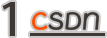### 前言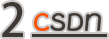### 机器学习

#### 2.1、什么是机器学习？

``````1, 3, 5, 7, 9
``````

#### 2.3、机器学习中的问题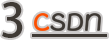### 线性回归和逻辑回归

#### 3.1、线性回归

``````1, 3, 5, 7, 9
``````

``````x, y
1, 1
2, 3
3, 5,
4, 7,
5, 9
``````

#### 3.2、线性回归实战

``````pip install scikit-learn
``````

``````import numpy as np
from sklearn.linear_model import LinearRegression
# 准备x的数据
X = np.array([
,
,
,
,

])
# 准备y的数据
y = np.array([1, 3, 5, 7, 9])
# 创建线性回归模块
lr = LinearRegression()
# 填充数据并训练
lr.fit(X, y)
# 输出参数
print("w=", lr.coef_, "b=", lr.intercept_)

``````

``````w= [2.] b= -1.0
``````

``````import numpy as np
from sklearn.linear_model import LinearRegression
X = np.array([
,
,
,
,

])
y = np.array([1, 3, 5, 7, 9])
lr = LinearRegression()
lr.fit(X, y)
y_predict = lr.predict(np.array([]))
print(y_predict)

``````

#### 3.3、逻辑回归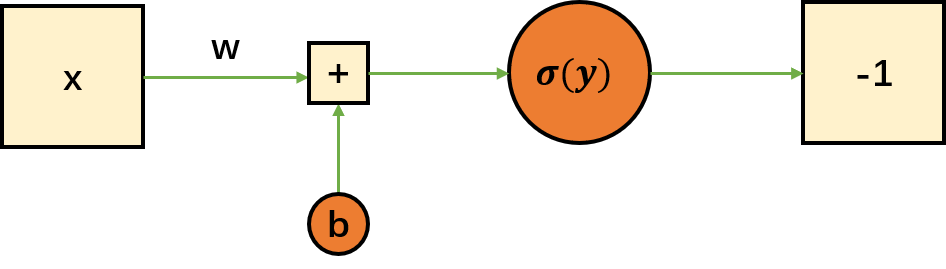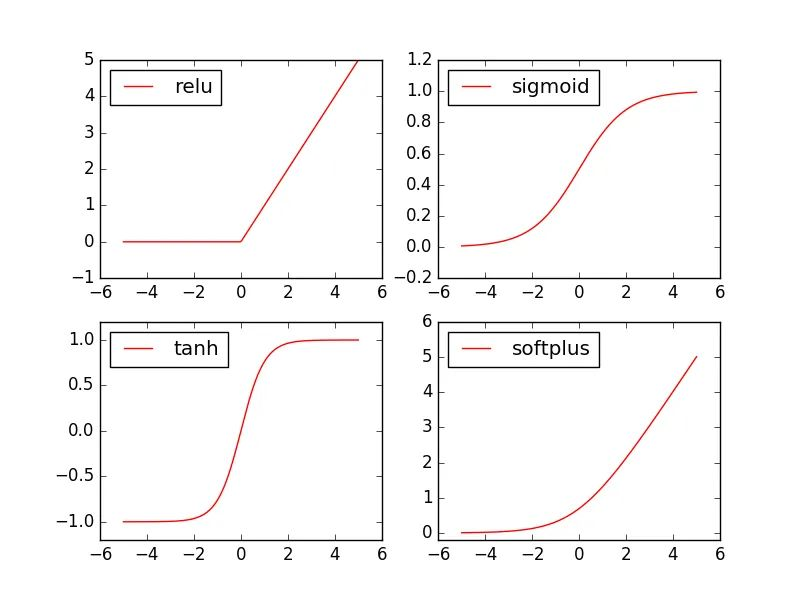#### 3.4、逻辑回归实战

``````import numpy as np
from sklearn.linear_model import LogisticRegression
# 准备X的数据
X = np.array([
,
,
,
,
,

])
# 准备y的数据
y = np.array([1, 0, 0, 1, 0, 1])
# 创建逻辑回归模型
lr = LogisticRegression()
# 填充数据并训练
lr.fit(X, y)
# 准备用于测试的数据
X_test = np.array([
,
,
,

])
# 判断测试数据是否及格
y_predict = lr.predict(X_test)
print(y_predict)

``````

``````[1 1 0 0]

``````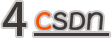### 神经网络

#### 4.1、神经网络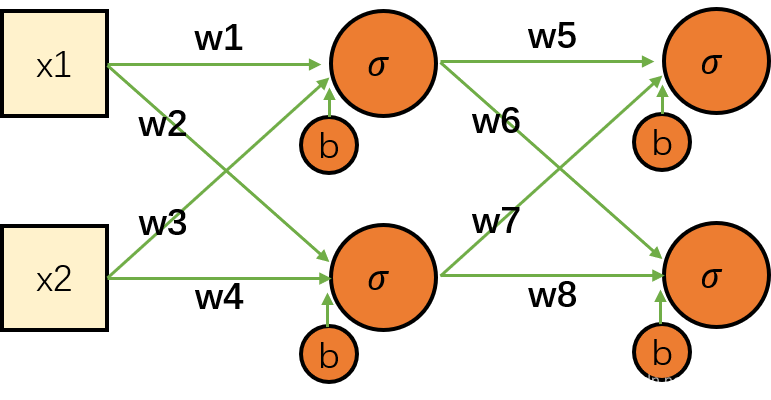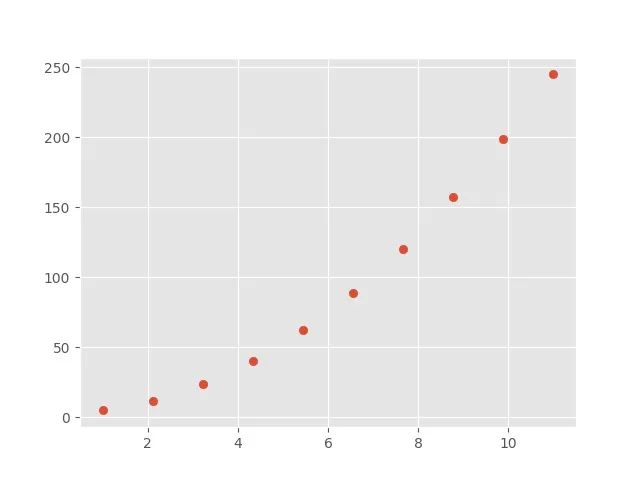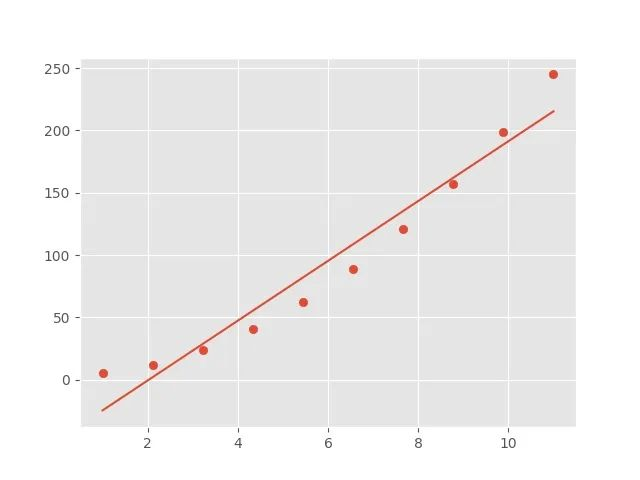#### 4.2、输入层、隐层、输出层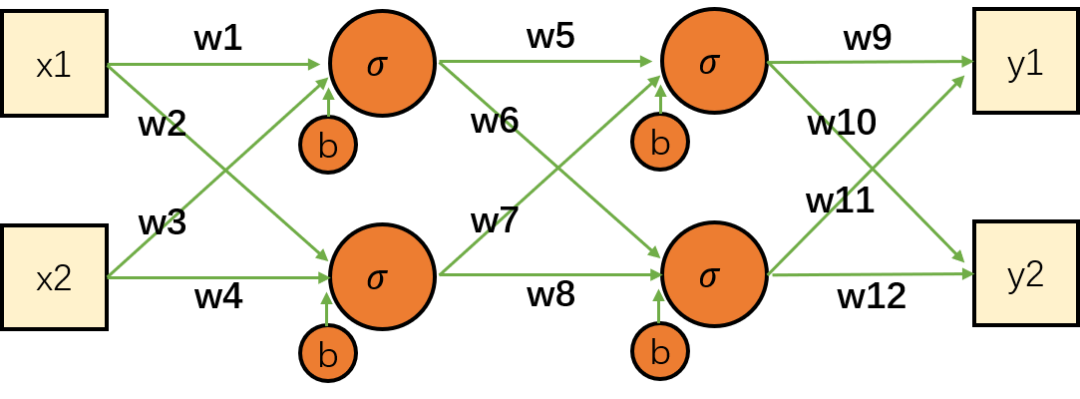#### 4.3、神经网络实战

``````from sklearn.datasets import load_iris
from sklearn.neural_network import MLPClassifier
from sklearn.model_selection import train_test_split

# 加载数据集
# 拆分数据集
X_train, X_test, y_train, y_test = train_test_split(iris_data['data'], iris_data['target'], test_size=0.25, random_state=1)
# 创建神经网络模型
mlp = MLPClassifier(solver='lbfgs', hidden_layer_sizes=[4, 2], random_state=0)
# 填充数据并训练
mlp.fit(X_train, y_train)
# 评估模型
score = mlp.score(X_test, y_test)
print(score)

``````

``````0.9210526315789473
``````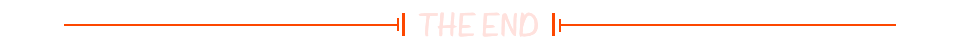2020-2021中国开发者调查报告重磅来袭，直接扫码或微信搜索「CSDN」公众号，后台回复关键词「开发者」，快速获取完整的报告内容！

``````
``````
``````☞时隔四年回归的澎湃芯片，能为雷军赌上一生荣耀的造车创业带来什么？☞那些跳槽做程序员的人，后悔了吗？☞不爱跳槽、月薪集中在 8K-17k、五成欲晋升为技术Leader|揭晓中国开发者真实现状☞Cortana 的谢幕，不一定是产品问题
``````20年前，《新程序员》创刊时，我们的心愿是全面关注程序员成长，中国将拥有新一代世界级的程序员。20年后的今天，我们有了新的使命：助力中国IT技术人成长，成就一亿技术人！